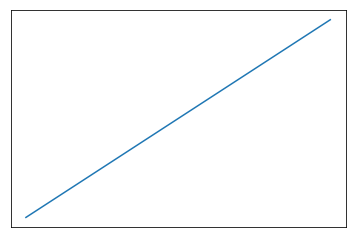# Customizing Tick and Label Style

Once you’ve got the correct strings printing along the axes, with as many (or few) ticks as you want, and at the right spacing, there are a lot of options for the way you can adjust the style of the rendering.

Let’s start with a simple graph.

%pylab inline

x = y = np.linspace(0, 10)

fig, ax = plt.subplots()
ax.plot(x, y)
Populating the interactive namespace from numpy and matplotlib

[<matplotlib.lines.Line2D at 0xa36c0b8>]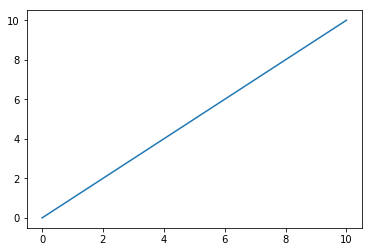## Changing Appearance

The tick_params() method for an Axis object gives you access to a whole bunch of options for formatting your ticks and their labels, including:

If the tick faces in or out

fig, ax = plt.subplots()
ax.plot(x, y)
ax.tick_params(axis='x',
direction='in')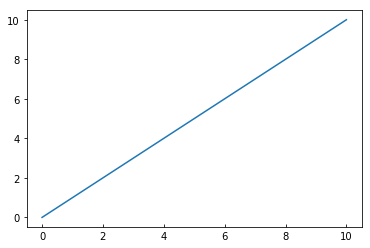How much the label is rotated

fig, ax = plt.subplots()
ax.plot(x, y)
ax.tick_params(axis='x',
rotation=90)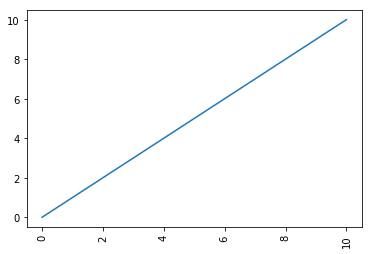How thick a tick is

fig, ax = plt.subplots()
ax.plot(x, y)
ax.tick_params(axis='x',
width=10)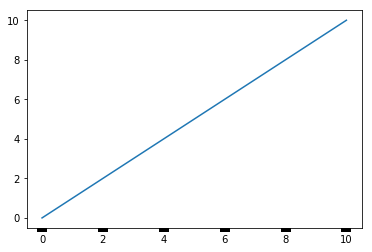The space between label and tick

fig, ax = plt.subplots()
ax.plot(x, y)
ax.tick_params(axis='x',
pad=20)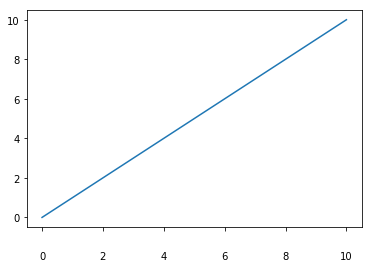The color of the ticks

fig, ax = plt.subplots()
ax.plot(x, y)
ax.tick_params(axis='x',
color='orange',
width=5)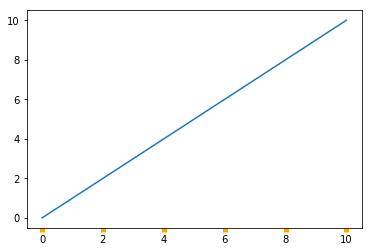## Hiding Them Altogether

We can also control whether or not the tick shows up

fig, ax = plt.subplots()
ax.plot(x, y)
ax.tick_params(axis='x',
bottom=False)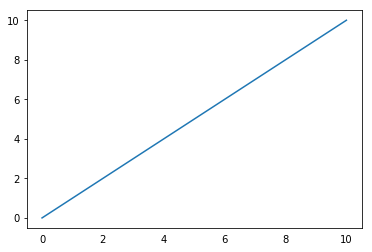Or the label

fig, ax = plt.subplots()
ax.plot(x, y)
ax.tick_params(axis='x',
labelbottom=False)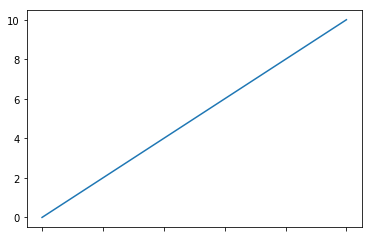Or all of it

fig, ax = plt.subplots()
ax.plot(x, y)
ax.tick_params(axis='both',
labelbottom=False,
bottom=False,
labelleft=False,
left=False)